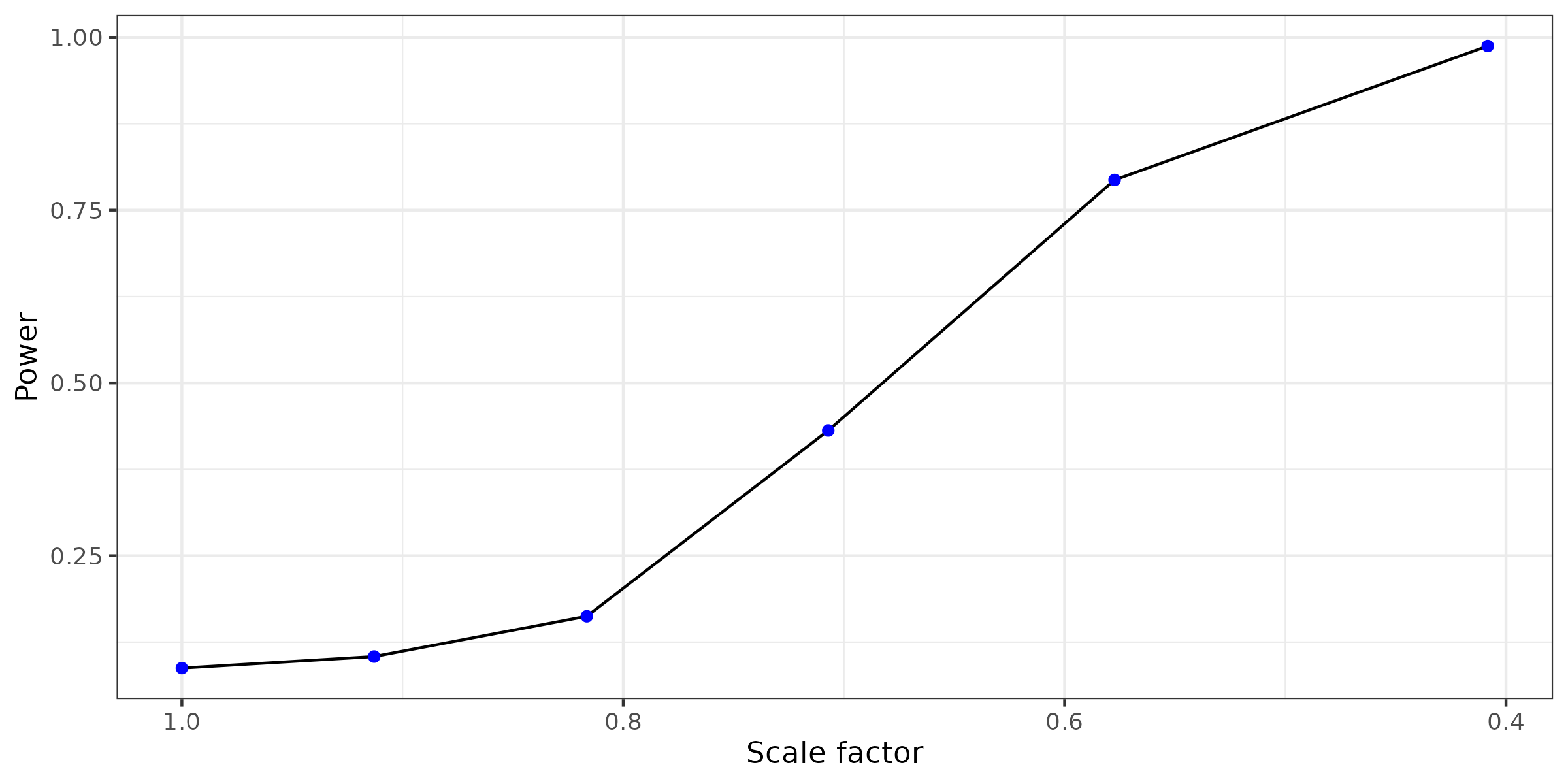# Hypervolume-Resampling

library(hypervolume)
library(palmerpenguins)
library(ggplot2)
library(gridExtra)
library(raster)
#> Loading required package: sp
data(penguins)
data(quercus)

# Introduction to Resampling Hypervolumes

When working with the package hypervolume, it is important to understand the statistical significance of the resulting hypervolume or hypervolumes. The methods introduced in this update are meant to characterize both variance from data sampling and variance due to non-deterministic behavior in the hypervolume algorithms.

The package provides the following functionalities:
- an interface for generating large resamples of hypervolumes
- methods for generating non-parametric confidence intervals for hypervolume parameters and null distributions for overlap statistics
- formal statistical tests based on hypervolumes

The purpose of this document is to provide use cases and explain best practices when using the new methods. The examples are chosen to highlight all the considerations that go into interpreting results.

## Use case 1: Effect of sample size on volume

The following code demonstrates how to visualize the effect of sample size on hypervolumes constructed using Gaussian kernels. We obtain climate data for Q. alba and Q. rubra by georeferencing the climate data at the geographical coordinates of each observation.

data("quercus")
data_alba = subset(quercus, Species=="Quercus alba")[,c("Longitude","Latitude")]
data_rubra = subset(quercus, Species=="Quercus rubra")[,c("Longitude","Latitude")]
climatelayers <- getData('worldclim', var='bio', res=10, path=tempdir())

# z-transform climate layers to make axes comparable
climatelayers_ss = climatelayers[[c(1,4,12,15)]]
for (i in 1:nlayers(climatelayers_ss))
{
climatelayers_ss[[i]] <- (climatelayers_ss[[i]] - cellStats(climatelayers_ss[[i]], 'mean')) / cellStats(climatelayers_ss[[i]], 'sd')
}
climatelayers_ss_cropped = crop(climatelayers_ss, extent(-150,-50,15,60))

# extract transformed climate values
climate_alba = extract(climatelayers_ss_cropped, data_alba)
climate_rubra = extract(climatelayers_ss_cropped, data_rubra)
combined_sample = data.frame(rbind(climate_rubra, climate_alba))
combined_sample["Species"] = quercus$Species # Create hypervolumes hv_alba = hypervolume(climate_alba) hv_rubra = hypervolume(climate_rubra) We first use hypervolume_resample with method = "boostrap seq" to define a sequence of sample sizes and resample 20 hypervolumes from each sample size. We then pass the output into hypervolume_funnel to visualize the results. To plot how a summary statistic describing a hypervolume varies with sample size, a function must be passed to the func field of hypervolume_funnel. A user inputted function must take a hypervolume object as an input and output a numeric. By default, func = get_volume. The confidence intervals in the plots are generated non-parametrically by taking quantiles at each sample size. When using hypervolume_funnel to plot the output of hypervolume_resample, a ggplot object is returned. It is then possible to add more plot elements to the result. alba_seq = hypervolume_resample("alba_seq", hv_alba, "bootstrap seq", n = 20, seq = seq(100, 1700, 400), cores = 32) rubra_seq = hypervolume_resample("rubra_seq", hv_rubra, "bootstrap seq", n = 20, seq = seq(100, 2100, 400), cores = 32) # Funnel Plots alba_plot = hypervolume_funnel(alba_seq) + geom_point(aes(y = upperq)) + geom_point(aes(y = lowerq)) + geom_point(aes(y = sample_mean), col = "blue") + theme_bw() + labs(title = "a)", subtitle = NULL) + ylab("Volume") + ylim(0, 0.8) + xlim(0, 2100) rubra_plot = hypervolume_funnel(rubra_seq) + geom_point(aes(y = upperq)) + geom_point(aes(y = lowerq)) + geom_point(aes(y = sample_mean), col = "blue") + theme_bw() + labs(title = "b)", subtitle = NULL) + ylab("Volume") + ylim(0, 0.8) + xlim(0, 2100) grid.arrange(alba_plot, rubra_plot, nrow = 1)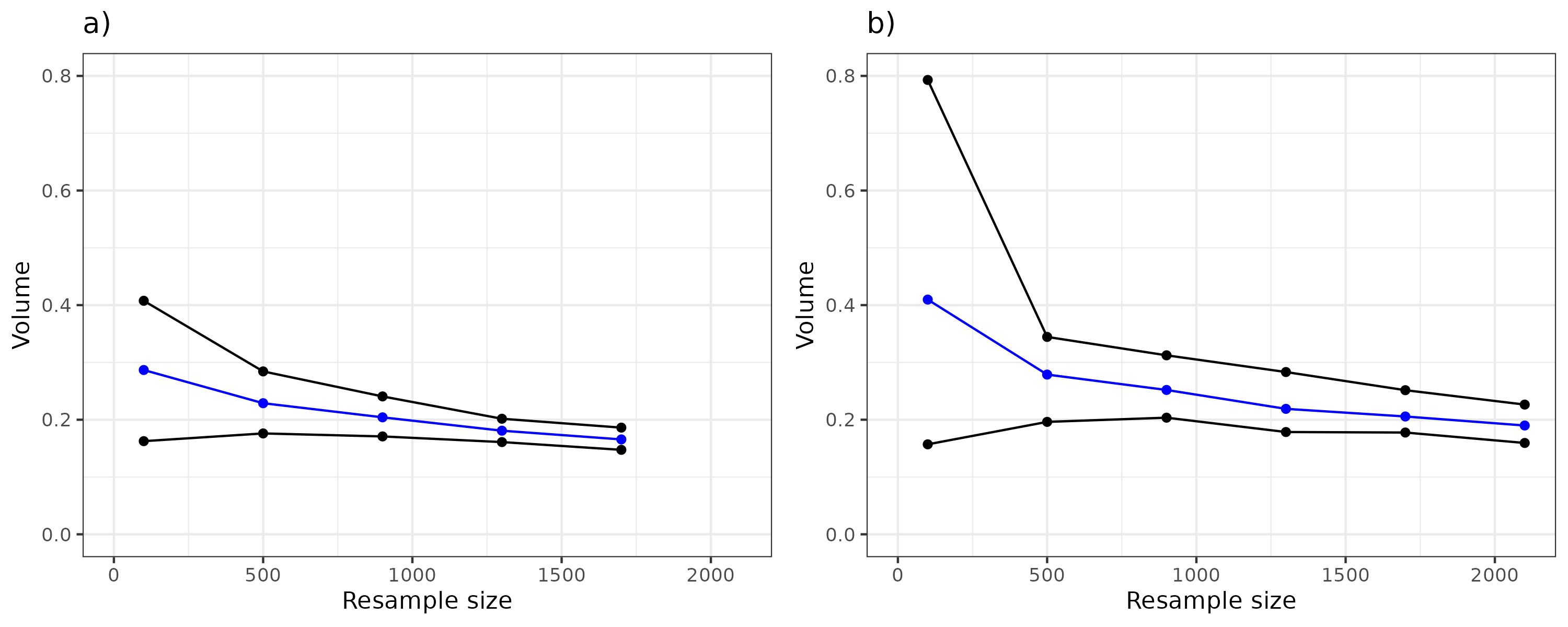The default contruction of hypervolumes uses kde.bandwidth = estimate_bandwidth(data, method = "silverman"). The above plot shows that volume decreases for both a) Q. alba and b) Q. rubra as sample size increases. This effect is due to Silverman bandwidth decreasing with sample size. In fact, Silverman bandwidth is not appropriate for multimodal data. The plot demonstrates this fact and shows that at small sample size, the hypervolume overestimates the true volume. Other methods for estimating bandwidth may be more accurate, but are computationally unfeasible for data with more than 3 dimensions. The estimated volume converges to the true volume of the population as sample size increases as shown in the above funnel plots. In the example, each confidence interval is a quantile of 20 resampled values. Improving the accuracy requires larger sample sizes which increases run time. It is recommended to use more cores to allow hypervolumes to be generated in parallel; however, by default, cores = 1 and the function runs sequentially. ## Use case 2: Effect of simulating bias when resampling Weights can be applied when resampling points so that the probability of sampling a particular point is proportional to the weight of that point. Weights are applied by either passing a list of weights to the weights field of hypervolume_resample, or by specifying the mu and sigma parameters. When using mu and sigma, the weight function is a multivariate normal density. mu is the mean of multivariate normal distribution while sigma is the diagonal covariance matrix of a multivariate normal distribution. cols_to_weigh specifies which columns to use as the input of the multivariate normal density. The following code demonstrates an application of applying weights while resampling data. In the example, we use quercus data to construct a hypervolume object from georeferenced climate data. For the sake of this example, consider a case of sampling Q. alba observations to the east of -75 longitude at half the rate of the other observations (imagine limited budget or time getting in the way of thorough sampling). We can apply resampling weights based on this prior knowledge and see if it significantly changes the distribution of the climate data. data("quercus") data_alba = subset(quercus, Species=="Quercus alba")[,c("Longitude","Latitude")] climatelayers <- getData('worldclim', var='bio', res=10, path=tempdir()) # z-transform climate layers to make axes comparable climatelayers_ss = climatelayers[[c(1,4,12,15)]] for (i in 1:nlayers(climatelayers_ss)) { climatelayers_ss[[i]] <- (climatelayers_ss[[i]] - cellStats(climatelayers_ss[[i]], 'mean')) / cellStats(climatelayers_ss[[i]], 'sd') } climatelayers_ss_cropped = crop(climatelayers_ss, extent(-150,-50,15,60)) # extract transformed climate values climate_alba = extract(climatelayers_ss_cropped, data_alba) # Generate Hypervolumes hv_alba = hypervolume(climate_alba,name='alba',samples.per.point=10) # Give all samples to the east of -75 longitude double the weight of all other observations to compensate for undersampling weights = ifelse(data_alba$Longitude > -75, 2, 1)

# Create new hypervolume using weighted bootstrapping
weighted_hv_alba = hypervolume_resample(hv = hv_alba, n=1, method = "weighted bootstrap", weights = weights, to_file = FALSE)
weighted_hv_alba = weighted_hv_alba[]

# Perform overlap test to see if the weights changed the distribution
alba_biased_combined_data = rbind(hv_alba@Data, weighted_hv_alba@Data)
alba_biased_combined_hv = hypervolume(alba_biased_combined_data)
alba_biased_combined_samples = hypervolume_resample("combined_biased_resample", hv = alba_biased_combined_hv, method = "bootstrap", n = 128, points_per_resample = nrow(alba_biased_combined_data)/2, verbose = FALSE, cores = 32)
result = hypervolume_overlap_test(hv_alba, weighted_hv_alba, alba_biased_combined_samples, cores = 32)

# Show null distribution and observed value
result$plots$sorensen +
theme_bw() +
labs(title = NULL) +
xlab("Sorensen Distance") +
ylab("Density")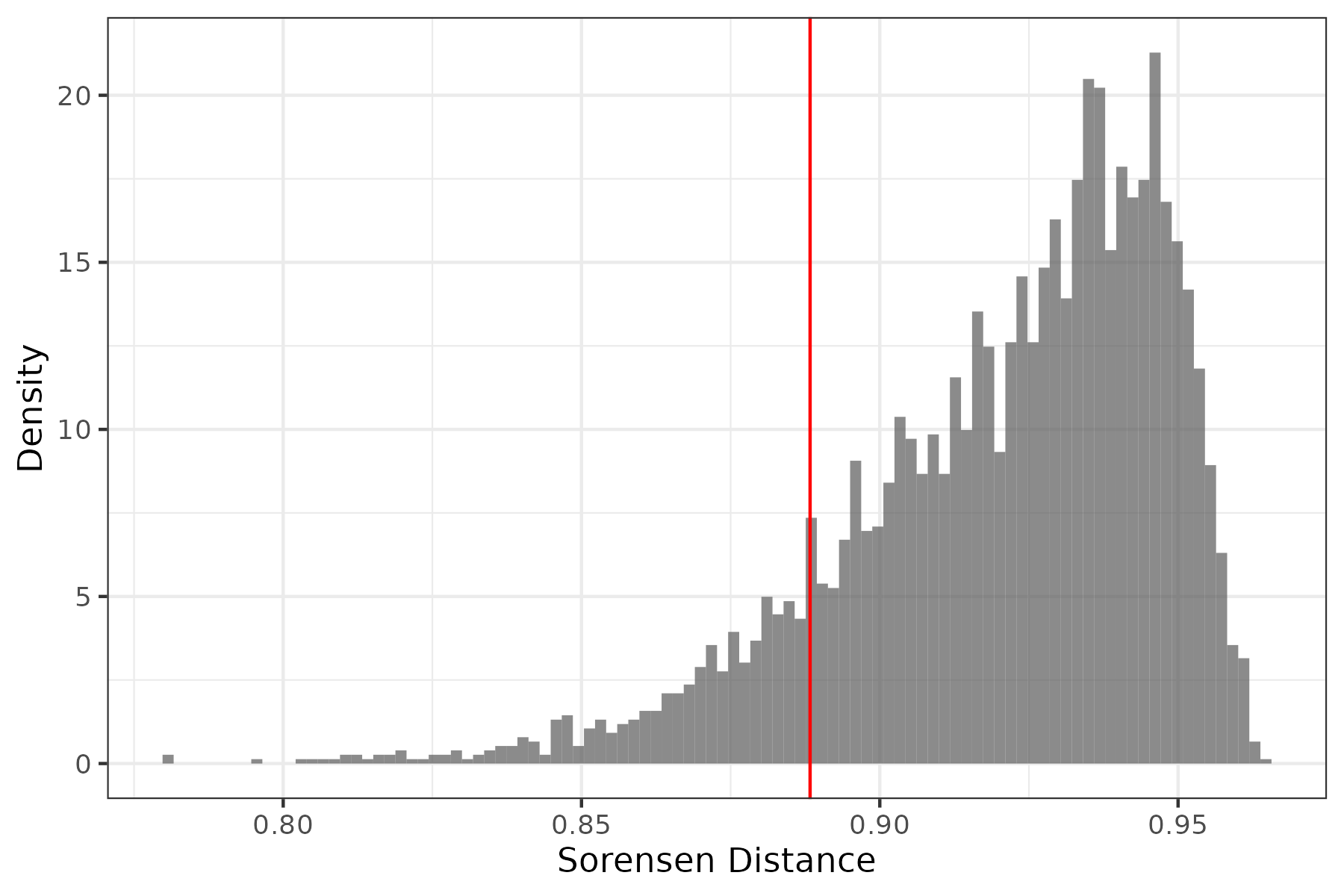The red line indicates the observed overlap statistic. Based on the above null distribution, p = 0.124. We cannot reject the null hypothesis that the climate hypervolume generated from the weighted bootstrap comes from a different distribution than the nonweighted hypervolume.

The following example demonstrates what happens when strong bias is applied to a small dataset. Using the palmerpenguin datatset, we want to induce a bias when resampling so that penguins with larger bills are resampled more often. We do this by using mu and sigma to define a multivariate normal distribution so that the resampling weight of each point is proportional to the normal density at that point.

hv = hypervolume(na.omit(penguins)[,3:6], verbose = FALSE)
cols_to_weigh = c("bill_length_mm", "bill_depth_mm")

# Highest weights are assigned to max bill length and max bill depth
mu = apply(hv@Data, 2, max)[cols_to_weigh]
sigma = apply(hv@Data, 2, var)[cols_to_weigh]*2
biased_path = hypervolume_resample("Bill bias", hv, method = "weighted bootstrap", n = 1, mu = mu, sigma = sigma, cols_to_weigh = cols_to_weigh)

# Read in hypervolume object from file

combined_dat = data.frame(rbind(hv@Data, biased_hv@Data))
combined_dat['Type'] = rep(c('original', 'biased'), each = nrow(hv@Data))
plot1 = ggplot(combined_dat) +
geom_histogram(aes(x = bill_depth_mm, fill = Type), bins = 20) +
facet_wrap(~Type) +
ggtitle("Distribution of Bill Depth") +
xlab("Bill depth (mm)") +
ylab("Count") +
theme_bw() +
theme(legend.position = "none")
plot2 = ggplot(combined_dat) +
geom_histogram(aes(x = bill_length_mm, fill = Type), bins = 20) +
facet_wrap(~Type) +
ggtitle("Distribution of Bill Length") +
xlab("Bill length (mm)") +
ylab(NULL) +
theme_bw() +
theme(legend.position = "none")
plot3 = ggplot(combined_dat) +
geom_histogram(aes(x = flipper_length_mm, fill = Type), bins = 20) +
facet_wrap(~Type) +
ggtitle("Distribution of Flipper Length") +
xlab("Flipper length (mm)") +
ylab("Count")+
theme_bw() +
theme(legend.position = "none")
plot4 = ggplot(combined_dat) +
geom_histogram(aes(x = body_mass_g, fill = Type), bins = 20) +
facet_wrap(~Type) +
ggtitle("Distribution of Body Mass") +
xlab("Body mass (g)") +
ylab(NULL) +
theme_bw() +
theme(legend.position = "none")
grid.arrange(plot1, plot2, plot3, plot4, nrow = 2)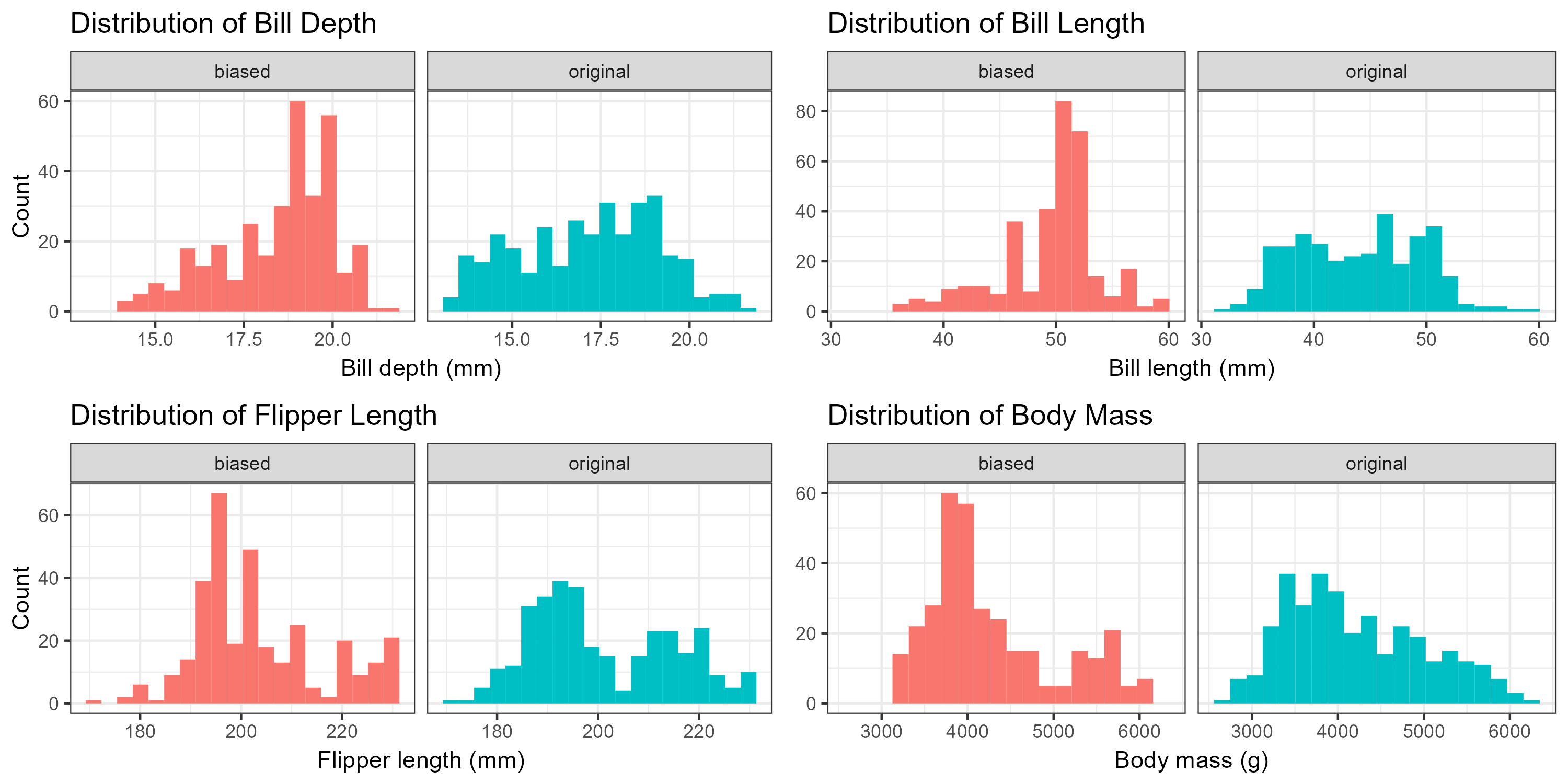The result shows that a bias is induced, but as a result, variance for all dimensions decrease as there are less unique points sampled. The volume will also be significantly reduced if the applied bias is strong. Therefore, it is recommended to only apply strong bias to larger datasets. In this example, sigma is chosen arbitrarily as twice the variance of the original columns. The larger sigma is, the weaker the bias and vice versa.

## Use case 3: Using overlap statistics to test for similarity of populations

The following code demonstrates how to test the null hypothesis that two samples come from the same distribution. In this example, we map the longitude and latitude data from quercus to a four dimensional climate space, as in demo(quercus).

To test whether the two species Quercus rubra and Quercus alba have the same climate niche, there are two approaches. In the first approach, we use the combined sample data as an approximation of the true distribution. To generate the null distribution for overlap statistics, we treat all of the data as having the same label and then bootstrap hypervolumes from the combined data. The overlaps of the resampled hypervolumes are used to generate the distribution of the overlap statistics. If the size of the two samples is the same, the function takes half the hypervolumes and overlaps them with each of the other hypervolumes. In this case, since the number of samples of Quercus rubra and Quercus alba are different, we need to bootstrap an equal number of hypervolumes for each sample size.

The second approach is a permutation test. For this method, the labels of the data are rearranged then the data is split by label. A pair of hypervolumes are generated from each split and overlap statistics are generated from each pair.

The benefit of the first method is the ability to generate multiple overlap statistics per hypervolume. If both methods generate $$N$$ hypervolumes, the first method will generate $$\frac{N^2}{4}$$ overlap statistics while the second method will generate $$\frac{N}{2}$$ overlap statistics. Since hypervolume construction and overlap both can be non-deterministic processes, method one will account for more of the variance from generating the overlap. However, when sample size is small, the combined data may not be a good approximation of the population. In this case, it is better to use method two, because it does not make any assumptions about the population, and generating more hypervolumes is fast for smaller sample sizes.

data("quercus")
data_alba = subset(quercus, Species=="Quercus alba")[,c("Longitude","Latitude")]
data_rubra = subset(quercus, Species=="Quercus rubra")[,c("Longitude","Latitude")]
climatelayers <- getData('worldclim', var='bio', res=10, path=tempdir())

# z-transform climate layers to make axes comparable
climatelayers_ss = climatelayers[[c(1,4,12,15)]]
for (i in 1:nlayers(climatelayers_ss))
{
climatelayers_ss[[i]] <- (climatelayers_ss[[i]] - cellStats(climatelayers_ss[[i]], 'mean')) / cellStats(climatelayers_ss[[i]], 'sd')
}
climatelayers_ss_cropped = crop(climatelayers_ss, extent(-150,-50,15,60))

# extract transformed climate values
climate_alba = extract(climatelayers_ss_cropped, data_alba)
climate_rubra = extract(climatelayers_ss_cropped, data_rubra)

# Generate Hypervolumes
hv_alba = hypervolume(climate_alba,name='alba',samples.per.point=10)
hv_rubra = hypervolume(climate_rubra,name='rubra',samples.per.point=10)

# Method 1: 2hr runtime with 12 threads
combined_sample = rbind(climate_alba, climate_rubra)
population_hat = hypervolume(combined_sample)

# Create bootstrapped hypervolumes of both sample sizes
method1_path_size_1669 = hypervolume_resample("quercus_1669_boot", population_hat, "bootstrap", n = 100, cores = 12)
method1_path_size_2110 = hypervolume_resample("quercus_2110_boot", population_hat, "bootstrap", n = 100, cores = 12)

result1 = hypervolume_overlap_test(hv_alba, hv_rubra, c(method1_path_size_1669, method1_path_size_2110), cores = 12)

#Method 2: 9hr runtime with 12 threads
method2_path = hypervolume_permute("rubra_alba_permutation", hv1, hv2, n = 1357, cores = 12)

result2 = hypervolume_overlap_test(hv1, hv2, method2_path, cores = 2)

# Graphical Results of null sorensen statistic
plot1 = result1$plots$sorensen +
xlab("Sorensen distance") +
ylab("Density") +
ggtitle("a)") +
xlim(0.7, 1) +
theme_bw()
plot1 = result2$plots$sorensen +
xlab("Sorensen distance") +
ylab("Density") +
ggtitle("b)") +
xlim(0.7, 1) +
theme_bw()
grid.arrange(plot1, plot2, ncol=2)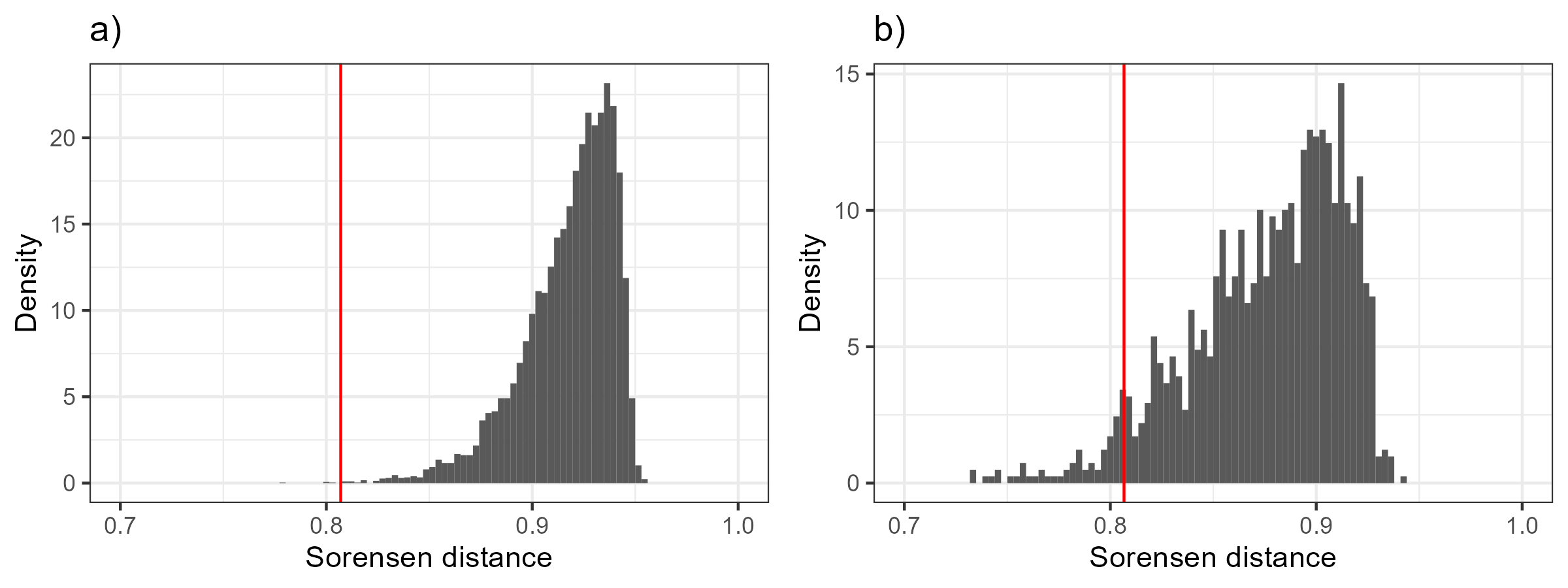For our example, the red line shows the observed value of the Sorensen distance. a) shows that the bootstrap overlap test results in a significantly lower p value than a significance level of 0.05. b) shows that the permutation overlap test also results in a low p value. Since p is less than 0.05 in both cases, we can reject the hypothesis that the two Quercus species have identical climate niches.

## Testing the power of the overlap test

The following code demonstrates how to test the power of the overlap test using simulated datasets. The power of a test is defined as the probability the test rejects the null hypothesis given that the alternative hypothesis is true. The first simulations quantify the power of the overlap test to reject the null hypothesis when two distributions have the same shape but are shifted. A sample dataset of N=20 observations in n=5 dimensions is drawn from a multivariate normal distribution with mean vector 0 and the identity covariance matrix. A second sample of M=40 observations is drawn from the same distribution with the mean shifted. The sorensen distance is used as the test statistic.

library(foreach)
library(mvtnorm)
library(doParallel)

# Choose number of threads to use for parallel computing

# Load required libraries in the environment of each thread and register cluster to parallel backend
clusterEvalQ(cl, {
library(hypervolume)
library(mvtnorm)
})
registerDoParallel(cl)

# Set shift distance and number of test replications
N = 40
M = 20
offset = # shift distance
reps = # Number of replications of the test

# Each replication takes around 4hrs. Total run time equals reps/nthreads * 4
# Returns a list of p values given by the overlap test
pvals = foreach(i = 1:reps, .combine = rbind) %dopar% {
# Random data N(0, I) and offset mean
x1 = rmvnorm(M, rep(0, 5))
x2 = rmvnorm(N, rep(offset, 5))
hv1 = hypervolume(x1)
hv2 = hypervolume(x2)
hv_combined = hypervolume(rbind(x1, x2))

path_M = hypervolume_resample(paste0("shift_", offset, "_M_", i), hv_combined, method = "bootstrap", n = 20, points_per_resample = M)
path_N = hypervolume_resample(paste0("shift_", offset, "_N_", i), hv_combined, method = "bootstrap", n = 20, points_per_resample = N)
result = hypervolume_overlap_test(hv1, hv2, c(path_M, path_N))
result$p_values } # Power is calculated as the percent of test replications that result in rejections which depends on the rejection threshold or alpha value. # We will use alpha = 0.05 power = mean(pvals[,"sorensen"] <= 0.05) After calculating the power at shift distances from 0 to 2.236, using 100 replications for each simulation, we can approximate the power of the overlap test as a function of shift distance. Note that the value when there is zero shift is the false positive rate. power0 = mean(pvals0[,"sorensen"] <= 0.05) power1 = mean(pvals1[,"sorensen"] <= 0.05) power2 = mean(pvals2[,"sorensen"] <= 0.05) power3 = mean(pvals3[,"sorensen"] <= 0.05) power4 = mean(pvals4[,"sorensen"] <= 0.05) power5 = mean(pvals5[,"sorensen"] <= 0.05) ggplot(data =NULL, aes(x = c(0, 0.4472136 0.8944272 1.3416408 1.7888544 2.2360680), y = c(power0, power1, power2, power3, power4, power5))) + geom_line() + theme_bw() + ylab("Power") + xlab("Shift distance")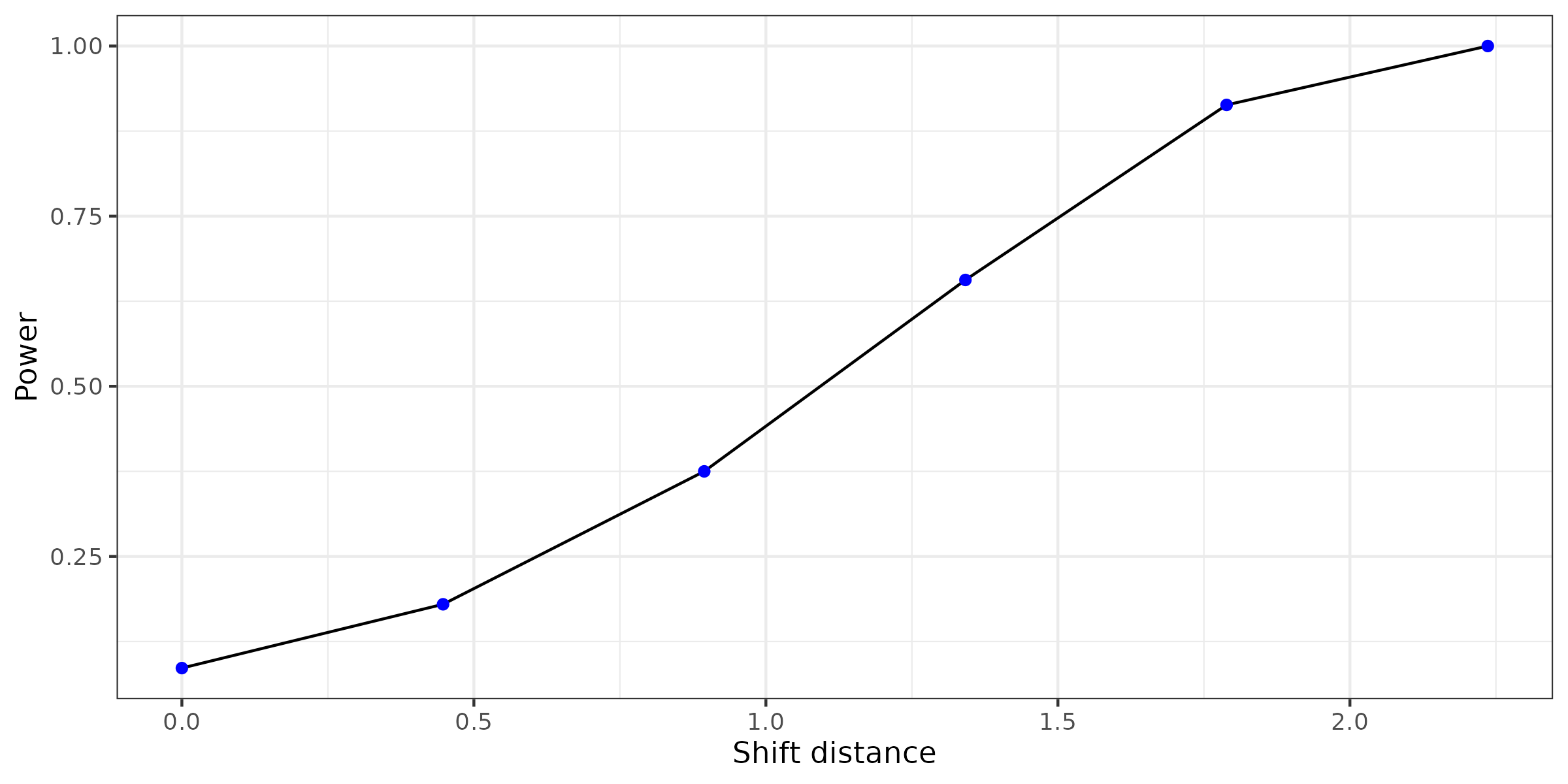The second simulations quantify the power of the overlap test to reject the null hypothesis when two distributions have the same mean but different shapes. A sample dataset of N=20 observations in n=2 dimensions is drawn from a multivariate normal distribution with mean vector 0 and the identity covariance matrix. A second sample of M=40 observations is drawn from the following distribution: $x_i^{(\alpha)} \sim N\left( \begin{bmatrix} 0 \\ 0 \\ \end{bmatrix}, \begin{bmatrix} \alpha^2 & 0 \\ 0 & \frac{1}{\alpha^2} \\ \end{bmatrix} \right)$ for $$\alpha =$$ 1, 0.913, 0.816, 0.707, 0.577, 0.408. The distributions for each value of $$\alpha$$ is visualized below.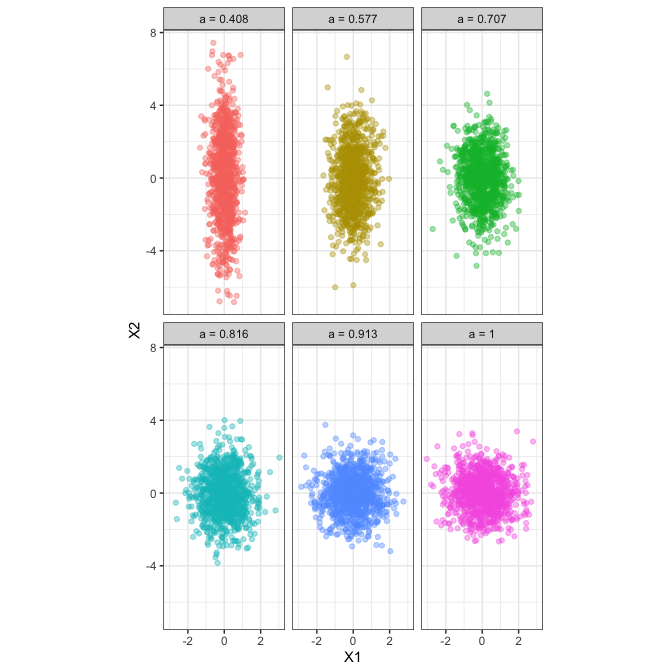N = 40 M = 20 offset = # Scale factor reps = # Number of replications of the test # Since the data is only 2 dimensions each replication only takes a few minutes pvals = foreach(i = 1:reps, .combine = rbind) %dopar% { x1 = rmvnorm(M, rep(0, 2)) x2 = rmvnorm(N, rep(0, 2), diag(c(offset^2, 1/(offset^2)))) hv1 = hypervolume(x1) hv2 = hypervolume(x2) hv_combined = hypervolume(rbind(x1, x2)) path_M = hypervolume_resample(paste0("scale_", offset, "_M_", i), hv_combined, method = "bootstrap", n = 20, points_per_resample = M) path_N = hypervolume_resample(paste0("scale_", offset, "_N_", i), hv_combined, method = "bootstrap", n = 20, points_per_resample = N) result = hypervolume_overlap_test(hv1, hv2, c(path_M, path_N)) result$p_values
}

Again we use sorensen distance as the test statistic to see how much the distributions overlap. After calculating the power at each scale factor using 150 replications for each simulation, we can approximate the power of the overlap test as a function of scale factor. Note that the value when scale factor is 1 is the false positive rate.

power0 = mean(pvals0[,"sorensen"] <= 0.05)
power1 = mean(pvals1[,"sorensen"] <= 0.05)
power2 = mean(pvals2[,"sorensen"] <= 0.05)
power3 = mean(pvals3[,"sorensen"] <= 0.05)
power4 = mean(pvals4[,"sorensen"] <= 0.05)
power5 = mean(pvals5[,"sorensen"] <= 0.05)

ggplot(data =NULL, aes(x = sqrt(1 - 0:5 * 1/6), y = c(power0, power1, power2, power3, power4, power5))) +
geom_line() +
theme_bw() +
ylab("Power") +
xlab("Scale factor") +
scale_x_reverse()